Related Articles

# Python | Pandas DataFrame.tz_localize

• Last Updated : 21 Feb, 2019

Pandas DataFrame is a two-dimensional size-mutable, potentially heterogeneous tabular data structure with labeled axes (rows and columns). Arithmetic operations align on both row and column labels. It can be thought of as a dict-like container for Series objects. This is the primary data structure of the Pandas.

Pandas` DataFrame.tz_localize()` function localize tz-naive index of a Series or DataFrame to target time zone. This operation localizes the Index.

Syntax: DataFrame.tz_localize(tz, axis=0, level=None, copy=True, ambiguous=’raise’, nonexistent=’raise’)

Parameter :
tz : string or pytz.timezone object
axis : the axis to localize
level : If axis ia a MultiIndex, localize a specific level. Otherwise must be None
copy : Also make a copy of the underlying data
ambiguous : When clocks moved backward due to DST, ambiguous times may arise
nonexistent : A nonexistent time does not exist in a particular timezone where clocks moved forward due to DST

Returns : Same type as the input.

Example #1: Use `DataFrame.tz_localize()` function to localize the given tz-naive index of the dataframe to the target timezone.

 `# importing pandas as pd``import` `pandas as pd`` ` `# Creating the DataFrame``df ``=` `pd.DataFrame({``'Weight'``:[``45``, ``88``, ``56``, ``15``, ``71``],``                   ``'Name'``:[``'Sam'``, ``'Andrea'``, ``'Alex'``, ``'Robin'``, ``'Kia'``],``                   ``'Age'``:[``14``, ``25``, ``55``, ``8``, ``21``]})`` ` `# Create the index``index_ ``=` `pd.date_range(``'2010-10-09 08:45'``, periods ``=` `5``, freq ``=``'H'``, tz ``=` `'US / Central'``)`` ` `# Set the index``df.index ``=` `index_`` ` `# Print the DataFrame``print``(df)`

Output :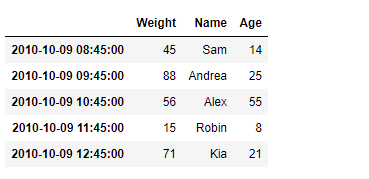Now we will use `DataFrame.tz_localize()` function to localize the given tz-naive index of the dataframe to the ‘Europe/Berlin’ timezone.

 `# Let's find out the current timezone``# of the given dataframe``print``(df.index)`` ` `# Let's localize the timezone of the``# dataframe index to 'Europe / Berlin'``df ``=` `df ``=` `df.tz_localize(tz ``=` `'Europe / Berlin'``)`` ` `# Let's find out the current timezone``# of the given dataframe``print``(df.index) `

Output :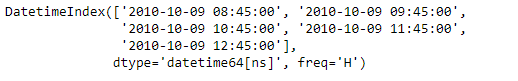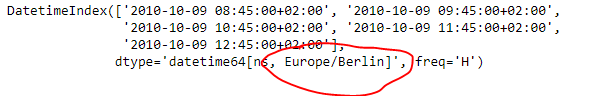As we can see in the output, the `DataFrame.tz_localize()` function has successfully localized the tz-naive index of the given dataframe to the target timezone.

Example #2 : Use `DataFrame.tz_localize()` function to localize the tz-naive Index of the given dataframe. The Index of the given dataframe is a MultiIndex.

 `# importing pandas as pd``import` `pandas as pd`` ` `# Creating the DataFrame``df ``=` `pd.DataFrame({``'Weight'``:[``45``, ``88``, ``56``, ``15``, ``71``],``                   ``'Name'``:[``'Sam'``, ``'Andrea'``, ``'Alex'``, ``'Robin'``, ``'Kia'``],``                   ``'Age'``:[``14``, ``25``, ``55``, ``8``, ``21``]})`` ` `# Create the MultiIndex``index_ ``=` `pd.MultiIndex.from_product([[``'Date'``], pd.date_range(``'2010-10-09 08:45'``, periods ``=` `5``, freq ``=``'H'``)],``           ``names ``=``[``'Level 1'``, ``'Level 2'``])`` ` `# Set the index``df.index ``=` `index_`` ` `# Print the DataFrame``print``(df)`

Output :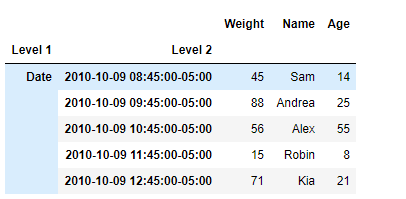Now we will use `DataFrame.tz_localize()` function to localize the tz-naive index of the given dataframe to ‘US/Central’.

 `# Let's find out the current timezone``# of the Level 1 of the given dataframe``print``(df.index[``1``])`` ` `# Let's localize the timezone of the``# level 1 of the dataframe to 'US / Central'``df ``=` `df.tz_localize(tz ``=` `'US / Central'``, level ``=` `1``)`` ` `# Let's find out the current timezone``# of the level 1 of the given dataframe``print``(df.index[``1``]) `

Output :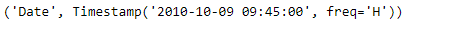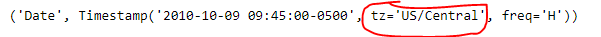As we can see in the output, the `DataFrame.tz_localize()` function has successfully localized the tz-naive index of the given dataframe to ‘US/Central’.

Attention geek! Strengthen your foundations with the Python Programming Foundation Course and learn the basics.

To begin with, your interview preparations Enhance your Data Structures concepts with the Python DS Course. And to begin with your Machine Learning Journey, join the Machine Learning – Basic Level Course

My Personal Notes arrow_drop_up